# 【统计学】概率计算

## 1.概率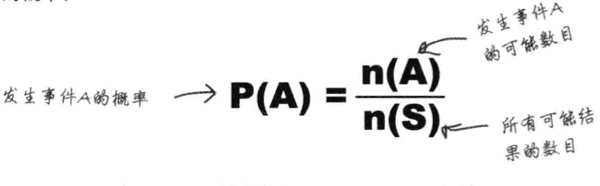## 2.维恩图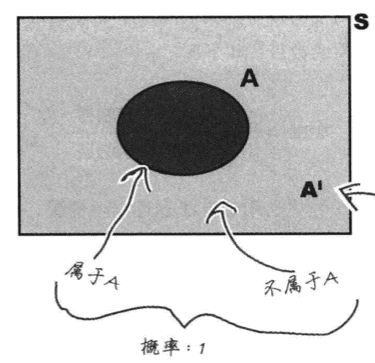P(A)+P(B)=1

## 5.条件概率

 同样，对于两个独立事件 A 与 B 有P(A B) = P(A)以及P(B A) = P(B)换句话说，如果 A 与 B 是相互独立的，那么 A 在 B 这个前提下的条件概率就是 A 自身的概率；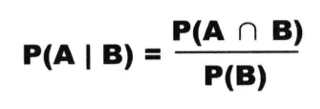## 6.概率树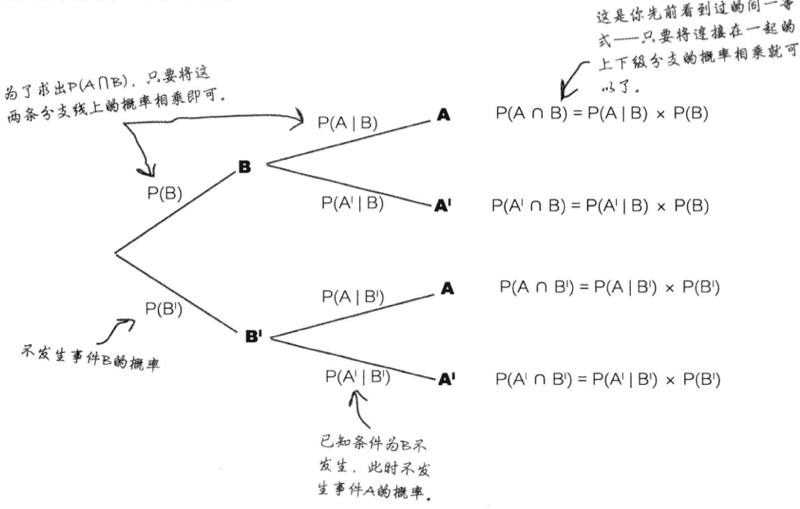## 7.全概率公式

 P(A)=P(A B1)*P(B1) + P(A B2)*P(B2) + … + P(A Bn)*P(Bn).

## 8.贝叶斯公式

 P(A B)=P(B A)*P(A)/P(B)
 贝叶斯定理由英国数学家贝叶斯 ( Thomas Bayes 1702-1763 ) 发展，用来描述两个条件概率之间的关系，比如 P(A B) 和 P(B A)。

### 贝叶斯理论及应用

 ▪ 贝叶斯分类算法 (应用:统计分析、测绘学) ▪ 贝叶斯风险 (应用:统计决策论) ▪ 贝叶斯公式 (应用:概率空间) ▪ 贝叶斯估计 (应用:参数估计) ▪ 贝叶斯区间估计 (应用:数学中的区间估计) ▪ 贝叶斯统计 (应用:统计决策论) ▪ 贝叶斯序贯决策函数 (应用:统计决策论) ▪ 经验贝叶斯方法 (应用:统计决策论)

 ▪ 贝叶斯定理 (应用:人工智能、心理学、遗传学) ▪ 贝叶斯分类器 (应用:模式识别、人工智能) ▪ 贝叶斯分析 (应用:计算机科学) ▪ 贝叶斯决策 (应用:人工智能) ▪ 贝叶斯逻辑 (应用:人工智能) ▪ 贝叶斯推理 (应用:数量地理学、人工智能) ▪ 贝叶斯网络 (应用:人工智能) ▪ 贝叶斯学习 (应用:模式识别)

 ▪ 贝叶斯主义 (应用:自然辩证法) ▪ 有信息的贝叶斯决策方法 (应用:生态系统生态学)

## 9.相关/独立事件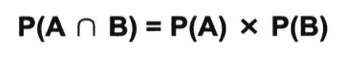## 11.独立性

 如果A和B相互独立，则P(A B) = P(A)